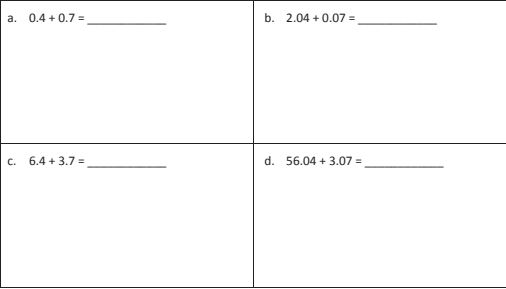Homework Explained - Math Practice 101Dear guest, you are not a registered member. As a guest, you only have read-only access to our books, tests and other practice materials.

As a registered member you can:

Registration is free and doesn't require any type of payment information. Click here to Register.
Go to page:
Lesson 9 Homework

•  a. 3 tenths + 4 tenths = tenths
•  b. 12 tenths + 9 tenths = tenths = one(s) tenth(s)
•  c. 3 hundredths + 4 hundredths = hundredths
•  d. 27 hundredths + 7 hundredths = hundredths = tenths hundredths
•  e. 4 thousandths + 3 thousandths = thousandths
•  f. 39 thousandths + 5 thousandths = thousandths = hundredths thousandths
•  g. 5 tenths + 7 thousandths = thousandths
•  h. 4 ones 4 tenths + 4 tenths = tenths
•  i. 8 thousandths + 6 ones 8 thousandths = thousandths
• Question 2

Solve using the standard algorithm.•  $$0.4+0.7=$$ $$2.04+0.07=$$ $$6.4+3.7=$$ $$56.04+3.07=$$

Yes, email page to my online tutor. (if you didn't add a tutor yet, you can add one here)